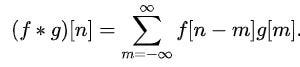Join thousands of students in our LangChain and Vector DBs in Production course, with over 50+ lessons and practical projects for FREE!.

## PublicationMathematics

# Convolution of Signals in 1-Dimension

Last Updated on May 22, 2020 by Editorial Team

#### Convolution is one of the most useful operators that finds its application in science, engineering, and mathematics

Convolution is a mathematical operation on two functions (f and g) that produces a third function expressing how the shape of one is modified by the other.

#### Convolution of discrete-time signals

A simple way to find the convolution of discrete-time signals is as shown

Input sequence x[n] = {1,2,3,4} with its index as {0,1,2,3}

Impulse response h[n] = {5,6,7,8} with its index as {-2,-1,0,1}

The blue arrow indicates the zeroth index position of x[n] and h[n]. The red pointer indicates the zeroth index position of the output convolved index. We can construct a table, as shown below. Multiply the elements of x and h as shown, and add them up diagonally.

`>> clc;  % clears the command window>> clear all; % clears all the variables in the workspace>> close all; % closes all the figure window`

Taking the input from the user

`>> % x[n] is the input discrete signal.>> x=input('Enter the input sequence x =');>> nx=input('Enter the index of the input sequence nx=');>> % h[n] is the impulse response of the system.>>h=input('Enter the impulse response of the system,second sequence h=');>> nh=input('Enter the index of the second sequence nh=');`

The output in the command window,

`Enter the input sequence x =[1 2 3 4]Enter the index of the input sequence nx=[0 1 2 3]Enter the impulse response of the system,second sequence h=[5 6 7 8]Enter the index of the second sequence nh=[-2 -1 0 1]`

Calculating the index of the convolved signal,

`>> % Index of the convolved signal>> n=min(nx)+min(nh):max(nx)+max(nh);`

Convolution

`>> y=conv(x,h);`

Display

`>> disp('The convolved signal is:');>> y>> disp('The index of convolved sequence is:');>> n`

The output in the command window,

`>> The convolved signal is:`
`y =`
`5    16    34    60    61    52    32`
`>> The index of convolved sequence is:`
`n =`
`-2    -1     0     1     2     3     4`

Let’s visualize these signals.

`>> subplot(311);>> stem(nx,x);>> subplot(312);>> stem(nh,h);>> subplot(313);>> stem(n,y);`

You can find the code on Github.

#### Convolution of continuous-time signals

`>> clc;>> clear all;>> close all;>> t=-3:0.01:8;>> x=(t>=-1 & t<=1); % pulse that exists for t>=-1 and t<=1>> subplot(311);>> plot(t,x);>> h1=(t>=1 & t<=3); % pulse that exists for t>=1 & t<=3>> h2=(t>3 & t<=4); % pulse that exists for t>3 & t<=4>> h=h1+(2*h2);>> subplot(312);>> plot(t,h);>> y=convn(x,h);>> y=y/100;>> t1=2*min(t):0.01:2*max(t);>> subplot(313);>> plot(t1,y);`

You can find code on Github.

#### Properties of Convolution

Convolution is a linear operator and has the following properties.

• The Commutative Property

x[n] * h[n] = h[n] * x[n] ( in discrete time )

x(t) * h(t) = h(t) * x(t) ( in continuous time )

• The Associative Property

x[n] * (h1[n] * h2[n]) = (x[n] * h1[n]) * h2[n] ( in discrete time )

x(t) * (h1(t) * h2(t)) = (x(t) * h1(t)) * h2(t) ( in discrete time )

• The Distributive Property

x[n] * (h1[n] + h2[n]) = (x[n] * h1[n]) + (x[n] * h2[n]) ( in discrete time )

x(t) * (h1(t) + h2(t)) = (x(t) * h1(t)) + (x(t) * h2(t)) ( in discrete time )

• Associativity with scalar multiplication

a(f * g) = (af) * g

for any real (or complex) number a

• Multiplicative identity
• Complex conjugation
• Relationship with differentiation
• Relationship with integration

#### Applications

Convolution finds its applications in many domains including digital image processing, digital signal processing, optics, neural networks, digital data processing, statistics, engineering, probability theory, acoustics, and many more.

#### ReferencesConvolution of Signals in 1-Dimension was originally published in Towards AI — Multidisciplinary Science Journal on Medium, where people are continuing the conversation by highlighting and responding to this story.

Published via Towards AI